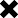### Long Term Capital Gain Tax Calculation Query

POSTED BY ON June 20, 2012 2:21 pm COMMENTS (3)

Hi Manish,
I am a regular reader of jagoinvestor blog and I appreciate you and
your team for their valuable efforts for providing investment and
money related information.
I have a few questions regarding Long Term Capital Gain. It would be
very nice if you can provide your valuable inputs on this matter.

Let me explain you the scenario:
I purchased a plot on 21st Feb 1991 at Rs 24265(Rs 22230 actual cost
of land and Rs 2035 registration charges). I sold the plot on 03rd
june 2011 at Rs 2066000.

Now I purchased a house on 31st octobeer 2011 at Rs 869400(Rs 800000
actual cost of house and Rs 69400 registration charges).
I am finding it difficult to calculate capital gain tax. I am not sure
whether to calculate my tax with indexation or without indexation so
as to reduce capital gain tax.

My questions regarding above scenario are as follows:
1. While calculating indexation does the total cost of plot(i.e. Rs
24265) will be considered or the actual cost of plot excluding
registration charges(i.e Rs 22230 only).
2. While calculating tax exemption on investment in new house does the
total cost of house(i.e. Rs 869400) will be considered or the actual
cost of house excluding registration charges(i.e Rs 800000 only).
3. I came across some blogs and found that exemption will be provided
on brokerage fees and other expenses. I would like to know that how
can I get it? Do I need to produce bills or proofs to get tax
exemption? I have gone through your previous article on capital gain
tax calculation.

I tried to calculate capital gain tax using your online calculator
also but it contains indexation details till 2009 only and there are
no fields provided in it to enter the investment details of other
property.

## 3 replies on this article “Long Term Capital Gain Tax Calculation Query”

1. Dear Amit, for LTCG calculation purpose the purchase price for you is 24265.

The purchase price of new house is 869400 Rs. for set off purpose against LTCG under section 54.

Dear Amit the CII for FY 2011-2012 was 785, so please use that for your LTCG calculation.

To support your claim for brok. & other fees, you need to have receipts of the same.

Thanks

Ashal

1. Amit Gupta says:

I found out that 20% tax rate will be applicable if capital gain is calculated with indexation and 10% tax rate will be applicable if capital gain is calculated without indexation.
Correct me if I do not have updated information.

1. So if I calculate my capital gain tax with indexation:

Indexed sale price = 24265 * 785/199 = Rs 95719
Capital Gain on sale = 2066000 – 95719 = Rs 1970281
Investment in new house = Rs 869400
Remaining Capital Gain = Rs 1100881
Tax on capital gain(20%) = Rs 220176.2

2. Now if I calculate my capital gain tax without indexation:

Actual sale price = Rs 24265
Capital Gain on sale = 2066000 – 24265 = Rs 2041735
Investment in new house = Rs 869400
Remaining Capital Gain = Rs 1172335
Tax on capital gain(10%) = Rs 117233.5

Can you tell that whether I have calculated the capital gain tax correctly or not?
If the above calculations are correct then I think my capital gain tax will be minimized if I calculate it without indexation.

1. Dear Amit, In case of capital gains from property. You have to calculate indexation compulsorily.

Thanks

Ashal

This site uses Akismet to reduce spam. Learn how your comment data is processed.